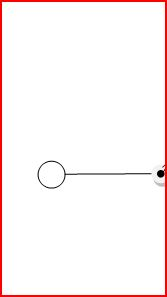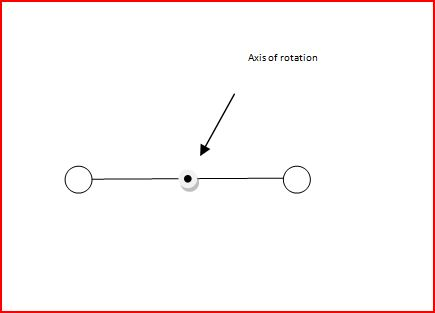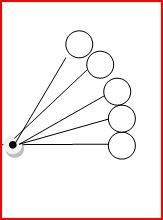# Moment of inertia and angular KE confusion

So the moment of inertia or a ring is MR2 I dont understand why. Here is my reasoning

Consider this shape (the ball is a point), the moment of inertia is MR2, there I agreebut now
what happens when you add another point on the other sidesince I = ΣMR2 then this is 2MR2

What about a ring or circle, that is nothing more than a bunch of points all the same distance from the centerso how many points are there? The circumference
so I= πR2MR2

I know that different objects moment of inertia differ by a number in front, like 1/2 or 3/5
Why is their no number prefix for Inertia in front of the formula for a hoop/ hollow cylinder/ circle that represents its circumference? How is this even derived?

Second question, How do you represent the angular Kinetic energy of this object?Left Point = A
Right point = B

½ I ωA2 + ⅓ I ωB2

½ I ( ωA2B2 )

both objects have same ω so

½ I 2ω2

Or

do I use ω to represent the angular velocity of the whole object and just keep it as

½ I ω2 with whatever I is from the previous question?

jbriggs444
Homework Helper
since I = ΣMR2 then this is 2MR2
You have two point-like balls of mass M at distance R. That's a total mass of M=2M. I = MR2.

but what about R, isn't their as many R's as there are points? Then where do the numbers in front of them moment of inertia come from? I how do you derive the MoI of a circle? Is my way correct? What about the second question?

jbriggs444
Homework Helper
but what about R, isn't their as many R's as there are points? Then where do the numbers in front of them moment of inertia come from? I how do you derive the MoI of a circle? Is my way correct? What about the second question?
For a collection mass or masses all at the same distance from the circle the "R" is the same for all.

For the second question... Just what is your second question?

For a collection mass or masses all at the same distance from the circle the "R" is the same for all.

For the second question... Just what is your second question?
the second half of my post, the KE

jbriggs444
Homework Helper
The second half of your post is confused. It mentions I without a formula for I. It uses ##\frac{1}{3} I \omega ^2## with no motivation. It mentions I using a single symbol for three distinct meanings.

I repeat: What is your question?

The second half of your post is confused. It mentions I without a formula for I. It uses ##\frac{1}{3} I \omega ^2## with no motivation. It mentions I using a single symbol for three distinct meanings.

I repeat: What is your question?

thats supposed to be 1/2
The formula for angular KE is 1/2 (moment of Inertia) (angular velocity)^2

but I got confused about what the MOI for that shape is supposed to be, and how to represent the angular velocity

How would you write the KE of that shape?
here was my reasoning

½ I ωA2 + ⅓ I ωB2

½ I ( ωA2+ωB2 )

both objects have same ω so

½ I 2ω2

Or

do I use ω to represent the angular velocity of the whole object and just keep it as

robphy
Homework Helper
Gold Member
First label individually...(assuming a common axis of rotation)
Particle A has rotational-KE: ##K_{rot,A}=\frac{1}{2}I_A \omega_A^2=\frac{1}{2}(m_Ar_A^2) \omega_A^2##
Particle B has rotational-KE: ##K_{rot,B}=\frac{1}{2}I_B \omega_B^2=\frac{1}{2}(m_Br_B^2) \omega_B^2##
The rotational-KE of the system ##K_{rot}=K_{rot,A}+K_{rot,B}##
Since the system is rigidly rotating, ##\omega_A=\omega_B## (simply call them ##\omega##).
I'm sure you can complete the story.

•David112234
jack action
Gold Member
how do you derive the MoI of a circle?
$$I = \int r^2 dm$$
source: https://en.wikipedia.org/wiki/Moment_of_inertia
Is my way correct?
Of course. You are using the sum instead of the integral, which is correct for a simple case:
$$I = \sum r^2\Delta m = r_A^2 m_A + r_B^2 m_B$$
if $r_A = r_B = r$ and $m_A + m_B = m$, then:
$$I = mr^2$$
$$E = E_A + E_B = \frac{1}{2}I_A \omega_A^2 + \frac{1}{2}I_B \omega_B^2 = \frac{1}{2}r_A^2 m_A \omega_A^2 + \frac{1}{2}r_B^2 m_B \omega_B^2$$
if $r_A = r_B = r$, $m_A + m_B = m$ and $\omega_A= \omega_B= \omega$, then:
$$E = \frac{1}{2}mr^2 \omega^2 = \frac{1}{2}I \omega^2$$
where $I = mr^2 = I_A + I_B$
•